# Fraction Calculator + Math PRO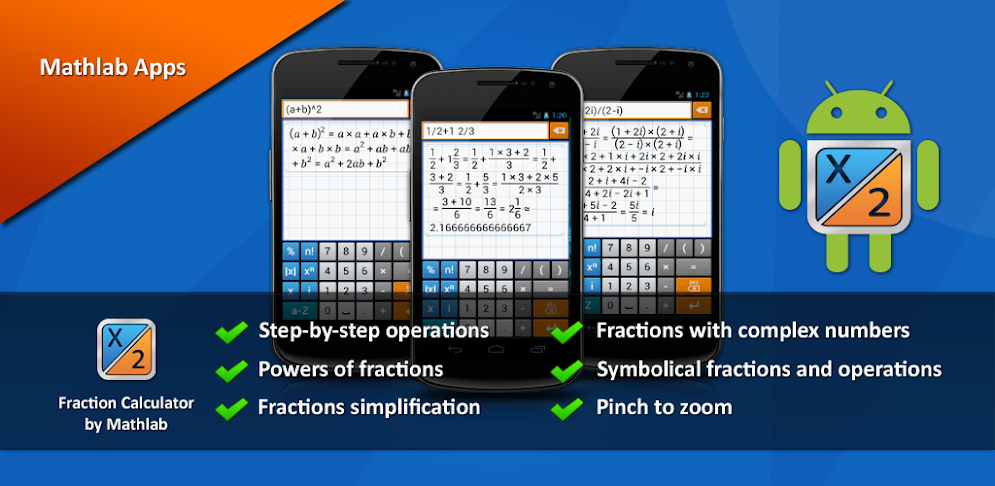## Description

Fraction calculator with step-by-step operations and algebra.
Shows results as you type.
Graphical display of expressions with history.
Use space to enter mixed number, e.g. 2 1/2
Help site with instructions and examples: http://frac.mathlab.us
If you have a question, send email to [email protected]

PRO FEATURES
* Workspaces to work on multiple tasks
* Store often used expressions in a library

FRACTION CALCULATOR
* Step-by-step operations (option to turn off)
* Arithmetic operations (+,-,*,/,÷), hold / to enter ÷
* Powers of fractions
* Fractions simplification
* Fractions with complex numbers
* Decimal to fraction conversion and back
* Symbolical fractions and operations
* Integer roots simplification
* Pinch to zoom

ALGEBRA
* Linear equations x+1=2 -> x=1
* Quadratic equations x^2-1=0 -> x=-1,1
* Approximate roots of higher polynomials
* Systems of linear equations, write one equation per line, x1+x2=1, x1-x2=2
* Polynomial long division
* Polynomial expansion, factoring
* Solving inequalities with one variable.
Double tap “(“to enter “less” sign.
Double tap “)” to enter “greater” sign.
* Linear and polynomial inequalities, x^3-4>4
* Inequalities with absolute values, abs(2x+3)<=5
* Compound inequalities, 1* Rational inequalities, (x+3)/(x-1)<=0

## Screenshots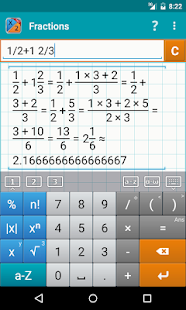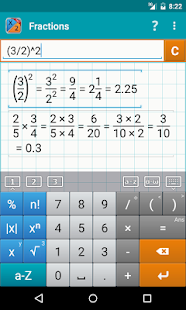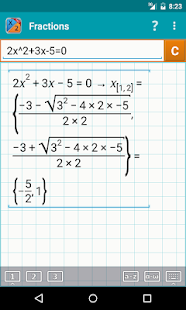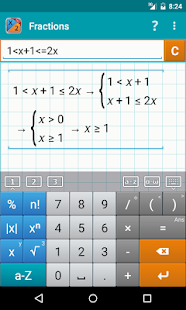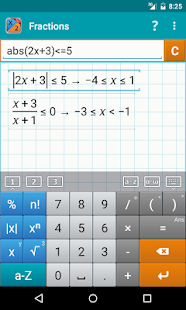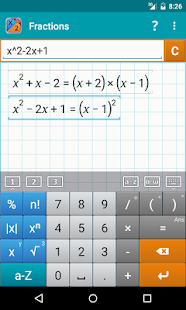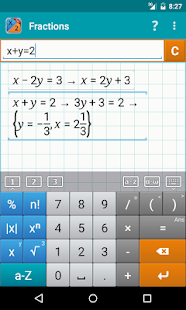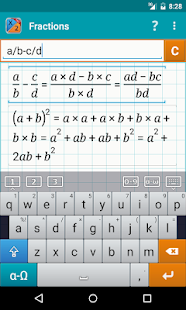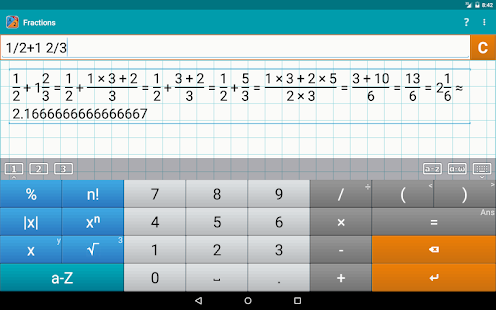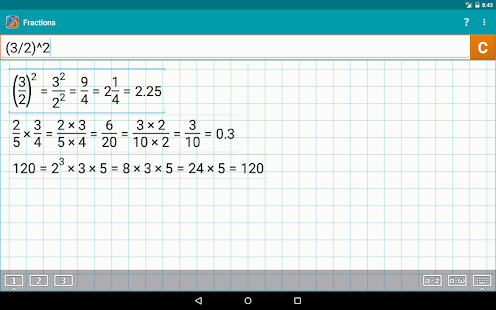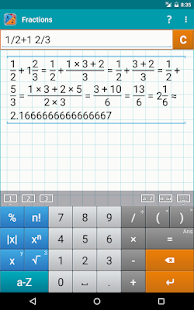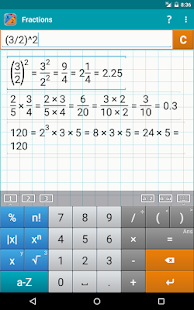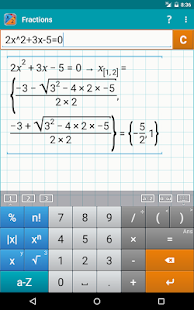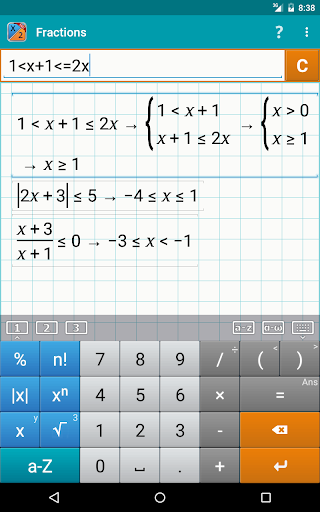Version 3.1.39 Uploader Hoster Heisenberg userscloud.comDownload Version 3.1.37 Uploader Hoster Heisenberg userscloud.comDownload Version 1.1.9 Uploader Hoster 200er uploaded.toDownload